Next: A Single-ended Method: Eigenvector-following Up: Optimisation of the Nudged Previous: Quenched Velocity Verlet Minimiser   Contents

## L-BFGS Minimiser

The BFGS algorithm belongs to the class of variable metric methods and is slightly different from the Davidon-Fletcher-Powell (DFP) method in the way in which the correction term is constructed  (see below). It has become generally recognised that the BFGS algorithm is superior to DFP in convergence tolerances, roundoff error and other empirical issues . The idea of the variable metric, or quasi-Newton, method is to build up a good approximation to the inverse Hessian matrix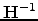by constructing a sequence of matrices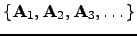with the following property: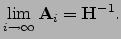(4.17)

If we are successful in doing so, combining two Newton-Raphson equations (see Equation 2.13) for consecutive iterations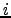and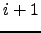, we find that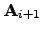should satisfy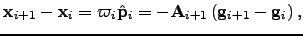(4.18)

where we have used the property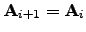, which must hold if both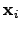and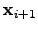are in the neighbourhood of minimum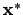.

At every iterationthe new approximation to the inverse Hessianshould be constructed in order to calculate the next step. The update formula must be consistent with Equation 2.18 and could take the form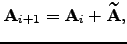(4.19)

where the correction term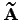is constructed using the gradient difference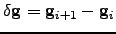, the step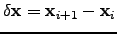, and the inverse Hessian matrix from the previous iteration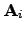. The BFGS update correction is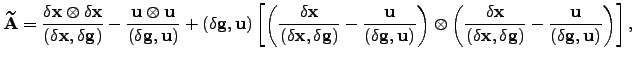(4.20)

where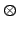denotes the direct product of two vectors, and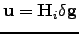.

Since at every iteration the old Hessian is overwritten with a new one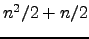storage locations are needed. This is an entirely trivial disadvantage over the conjugate gradient methods for any modest value of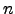. However, for large-scale problems it is advantageous to be able to specify the amount of storage BFGS is allowed to use.

There is a version of the BFGS algorithm that allows us to vary the amount of storage available to BFGS and is particularly suitable for large-scale problems . The difference between this limited memory BFGS (L-BFGS) algorithm and the standard BFGS is in the Hessian matrix update. L-BFGS stores each correction separately and the Hessian matrix is never formed explicitly. To store each correction to the Hessian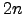storage locations are needed . The user can specify the number of corrections L-BFGS is allowed to store. Every iteration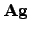is computed according to a recursive formula described by Nocedal . For the first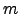iterations L-BFGS is identical to the BFGS method. After the firstiterations the oldest correction is discarded. Thus, by varyingthe iteration cost can also be controlled, which makes the method very efficient and flexible. In general, because onlyrecent corrections are stored L-BFGS is better able to use additional storage to accelerate convergence. Here we employed a modified version of Nocedal's L-BFGS implementation  in which the line search was removed and the maximum step size was limited for each image separately.Next: A Single-ended Method: Eigenvector-following Up: Optimisation of the Nudged Previous: Quenched Velocity Verlet Minimiser   Contents
Semen A Trygubenko 2006-04-10